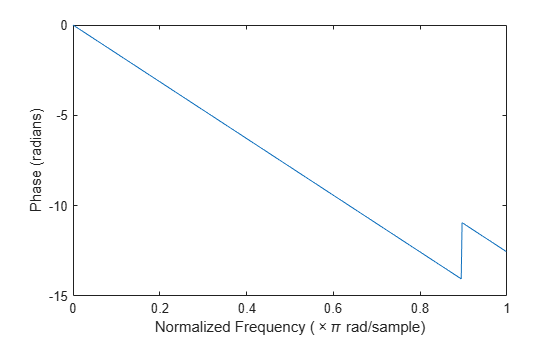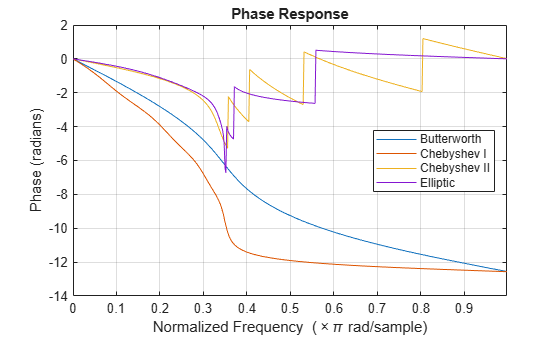Documentation

islinphase

Determine whether filter has linear phase

Syntax

flag = islinphase(b,a)
flag = islinphase(sos)
flag = islinphase(d)
flag = islinphase(...,tol)
flag = islinphase(h)

Description

flag = islinphase(b,a) returns a logical output, flag, equal to true if the filter coefficients in b and a define a linear phase filter. flag is equal to false if the filter does not have linear phase.

flag = islinphase(sos) returns true if the filter specified by second order sections matrix, sos, has linear phase. sos is a K-by-6 matrix, where the number of sections, K, must be greater than or equal to 2. Each row of sos corresponds to the coefficients of a second order (biquad) filter. The ith row of the sos matrix corresponds to [bi(1) bi(2) bi(3) ai(1) ai(2) ai(3)].

flag = islinphase(d) returns true if the digital filter, d, has linear phase. Use designfilt to generate d based on frequency-response specifications.

flag = islinphase(...,tol) uses the tolerance, tol, to determine when two numbers are close enough to be considered equal. If not specified, tol, defaults to eps^(2/3).

flag = islinphase(h) determines if the dfilt filter object h has linear phase.

Examples

collapse all

Use the window method to design a tenth-order lowpass FIR filter with normalized cutoff frequency 0.55. Verify that the filter has linear phase.

d = designfilt('lowpassfir','DesignMethod','window', ...
'FilterOrder',10,'CutoffFrequency',0.55);
flag = islinphase(d)
flag = logical
1

[phs,w] = phasez(d);

plot(w/pi,phs)
xlabel('Frequency \omega/\pi')
ylabel('Phase')IIR filters in general do not have linear phase. Verify the statement by constructing eighth-order Butterworth, Chebyshev, and elliptic filters with similar specifications.

ord = 8;
Wcut = 0.35;
atten = 20;
rippl = 1;

[zb,pb,kb] = butter(ord,Wcut);
sosb = zp2sos(zb,pb,kb);

[zc,pc,kc] = cheby1(ord,rippl,Wcut);
sosc = zp2sos(zc,pc,kc);

[zd,pd,kd] = cheby2(ord,atten,Wcut);
sosd = zp2sos(zd,pd,kd);

[ze,pe,ke] = ellip(ord,rippl,atten,Wcut);
sose = zp2sos(ze,pe,ke);

Plot the phase responses of the filters. Determine whether they have linear phase.

fv = fvtool(sosb,sosc,sosd,sose,'Analysis','phase');
legend(fv,'Butterworth','Chebyshev I','Chebyshev II','Elliptic')phs = [islinphase(sosb) islinphase(sosc) ...
islinphase(sosd) islinphase(sose)]
phs = 1x4 logical array

0   0   0   0